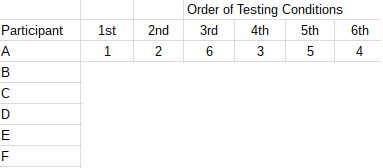# Latin Square Design: Definition and Balanced Latin Square Algorithm

## What is a Latin Square?

A Latin square is an ancient puzzle where you try to figure out how many ways Latin letters can be arranged in a set number of rows and columns (a matrix); each symbol appears only once in each row and column. It’s called a Latin square because it was developed based on Leonard Euler’s works, which used Latin symbols. However, any letters can be used.

## What is a Balanced Latin Square Design?

While a Latin square ensures that each letter appears an equal number of times, it doesn’t protect against order effects. For example, in the above 3 x 3 example square, treatment B follows A three times (in the rows). It follows C zero times. A balanced Latin square evens out the what-follows-what scenario, protecting against order effects.

If you have an even number of experimental conditions, constructing the square is very easy.

## Making the Square

The following algorithm can be used to make a balanced square for an even number of testing conditions:

## Example

Make a balanced square design for six participants A B C D E F with six testing conditions.

Step 1: Make the first row using the formula: row1 = 1,2,n,3,n-1,n-2.Step 2: Continue filling in the columns sequentially until the square is completed.A completed balanced square design with an even number of conditions.

For an odd number of conditions, making the balanced square becomes a lot more complicated (the experiment will also likely become more time consuming and expensive). Each participant must be tested twice for each condition, doubling the number of treatments given. For example, if you have 5 treatments, participant A might be given {1,2,5,3,4,3,2,1,5,4}.

References:
Experimental Psychology
Eakta Jain. Order Effects. University of Florida.

CITE THIS AS:
Stephanie Glen. "Latin Square Design: Definition and Balanced Latin Square Algorithm" From StatisticsHowTo.com: Elementary Statistics for the rest of us! https://www.statisticshowto.com/latin-square-design/